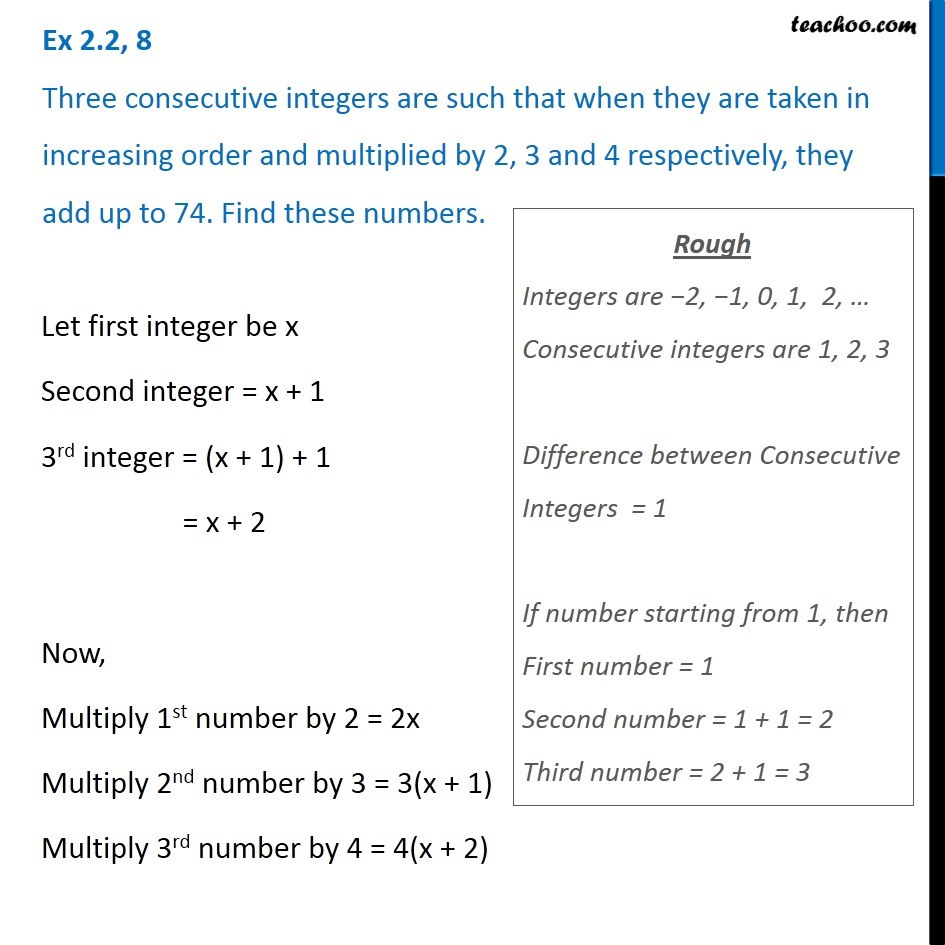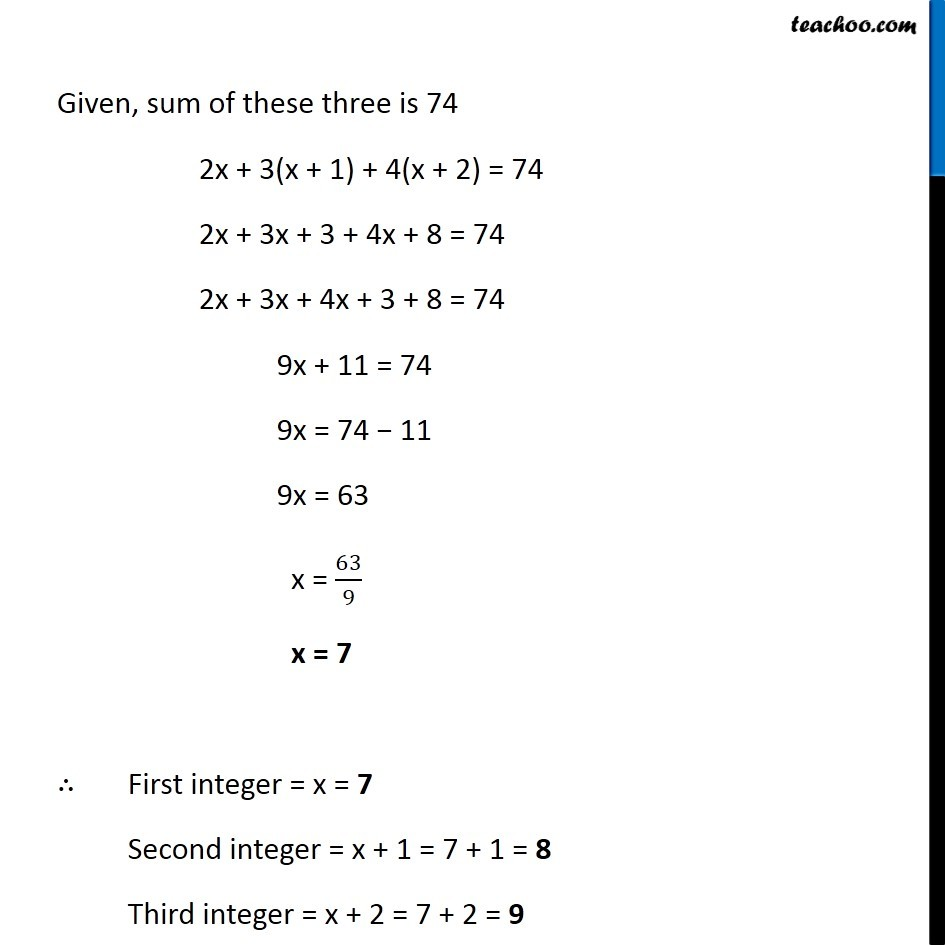Solving easy equations - Word Problems

Chapter 2 Class 8 Linear Equations in One Variable
Serial order wiseLearn in your speed, with individual attention - Teachoo Maths 1-on-1 Class

### Transcript

Question 8 Three consecutive integers are such that when they are taken in increasing order and multiplied by 2, 3 and 4 respectively, they add up to 74. Find these numbers.Let first integer be x Second integer = x + 1 3rd integer = (x + 1) + 1 = x + 2 Now, Multiply 1st number by 2 = 2x Multiply 2nd number by 3 = 3(x + 1) Multiply 3rd number by 4 = 4(x + 2) Rough Integers are −2, −1, 0, 1, 2, … Consecutive integers are 1, 2, 3 Difference between Consecutive Integers = 1 If number starting from 1, then First number = 1 Second number = 1 + 1 = 2 Third number = 2 + 1 = 3 Given, sum of these three is 74 2x + 3(x + 1) + 4(x + 2) = 74 2x + 3x + 3 + 4x + 8 = 74 2x + 3x + 4x + 3 + 8 = 74 9x + 11 = 74 9x = 74 − 11 9x = 63 x = 63/9 x = 7 ∴ First integer = x = 7 Second integer = x + 1 = 7 + 1 = 8 Third integer = x + 2 = 7 + 2 = 9